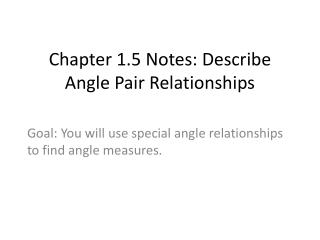DownloadDownload PresentationChapter 1.5 Notes: Describe Angle Pair Relationships

# Chapter 1.5 Notes: Describe Angle Pair Relationships

Télécharger la présentation## Chapter 1.5 Notes: Describe Angle Pair Relationships

- - - - - - - - - - - - - - - - - - - - - - - - - - - E N D - - - - - - - - - - - - - - - - - - - - - - - - - - -
##### Presentation Transcript

1. Chapter 1.5 Notes: Describe Angle Pair Relationships Goal: You will use special angle relationships to find angle measures.

2. Two angles are complementary angles if the sum of their measures is 90o. • Each angle is the complement of the other. • Two angles are supplementary angles if the sum of their measures is 180o. • Each angle is the supplement of the other.

3. Complementary angles and supplementary angles can be adjacent angles or nonadjacent angles. • Adjacent angles are two angles that share a common vertex and side, but have no common interior points.

4. Ex.1: In the figure, name a pair of complementary angles, a pair of supplementary angles, and a pair of adjacent angles. Ex.2: In the figure, name a pair of complementary angles, a pair of supplementary angles, and a pair of adjacent angles.

5. Ex.3: a. Given that is a complement of and , find . b. Given that is a supplement of and , find .

6. Ex.4: When viewed from the side, the frame of a ball-return net forms a pair of supplementary angles with the ground. Find and .

7. Ex.5: and are complementary angles. Find the measures of the angles if and .

8. Ex.6: Two roads intersect to form supplementary angles, and . Find and .

9. Angle Pairs: • Two adjacent angles are a linear pairif their noncommon sides are opposite rays. • The angles in a linear pair are supplementary angles. • Two angles are vertical angles if their sides form two pairs of opposite rays.

10. Ex.7: Identify all of the linear pairs and all of the vertical angles in the figure below. Ex.8: Two angles form a linear pair. The measure of one angle is 5 times the measure of the other. Find the measure of each angle.

11. Ex.9: The measure of an angle is twice the measure of its complement. Find the measure of each angle. Ex.10: Two angles form a linear pair. The measure of one angle is 3 times the measure of the other angle. Find the measure of each angle. Ex.11: Two angles form a linear pair. The measure of one angle is 5o more than the other angle. Find the measure of each angle.